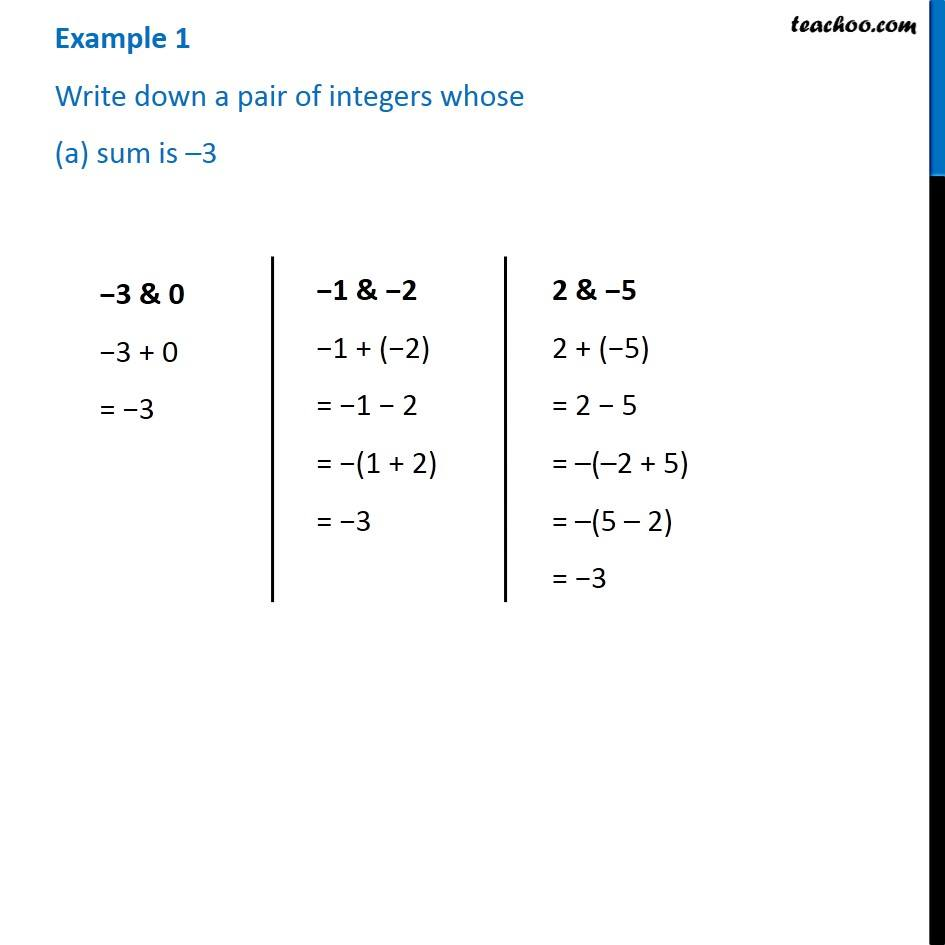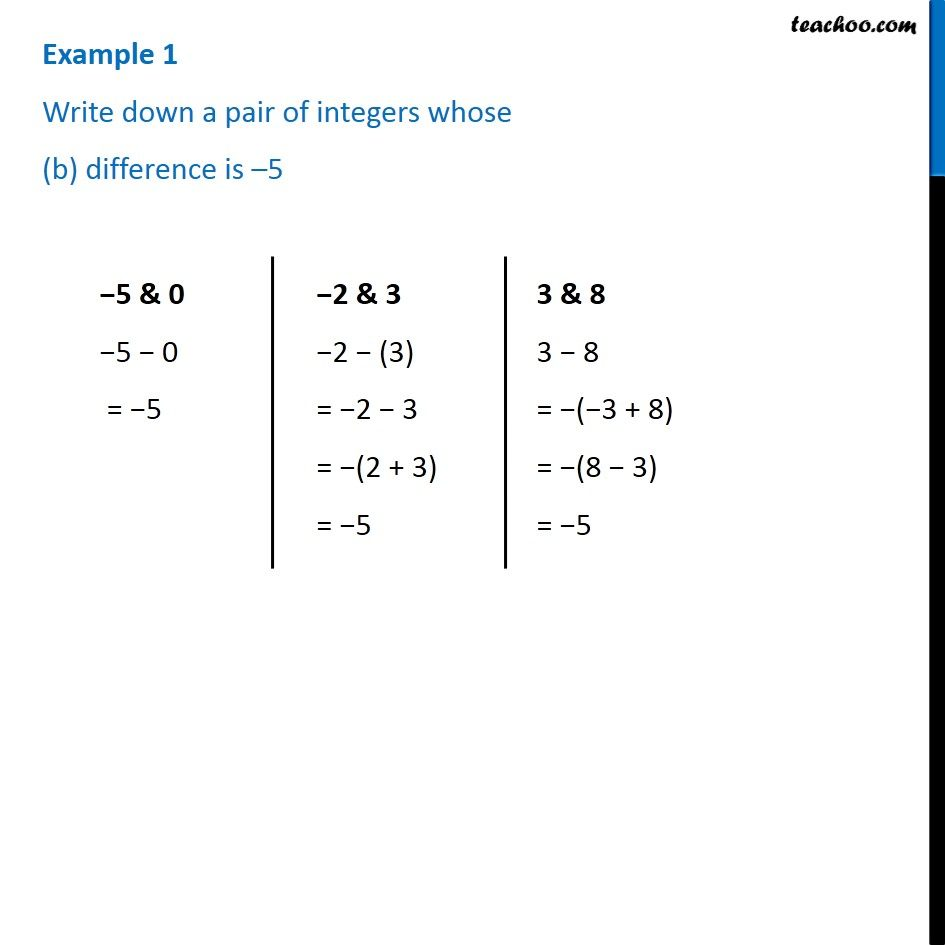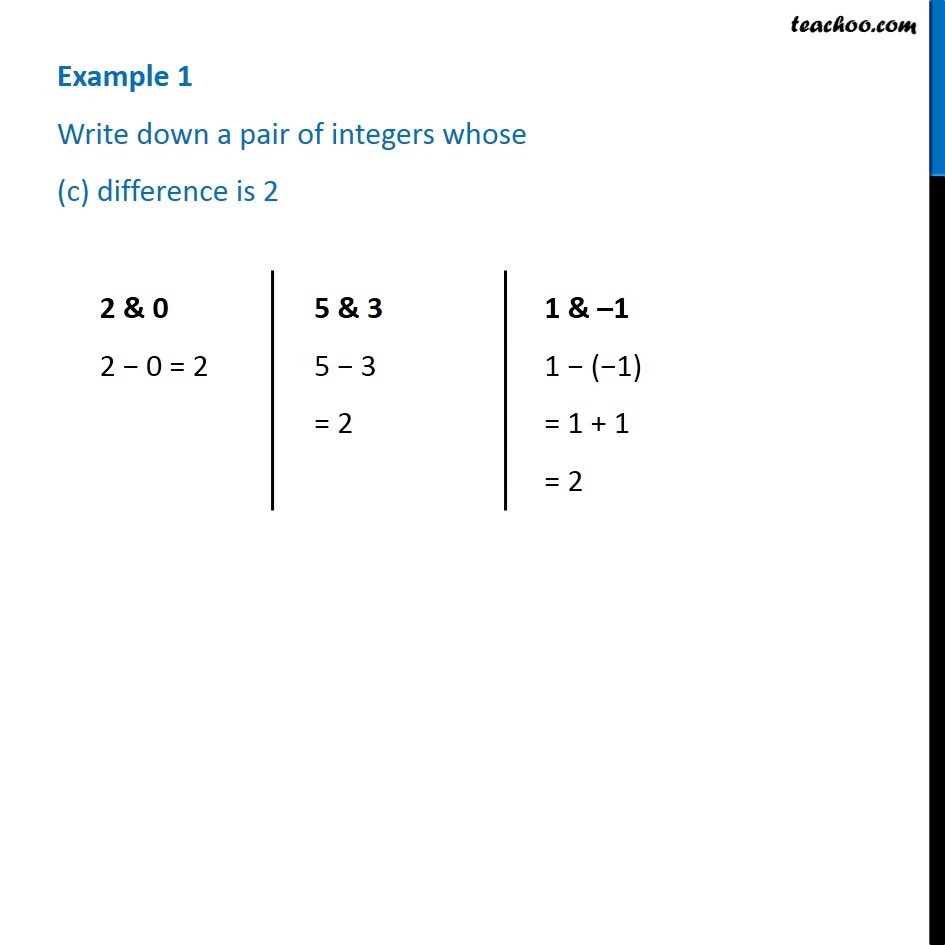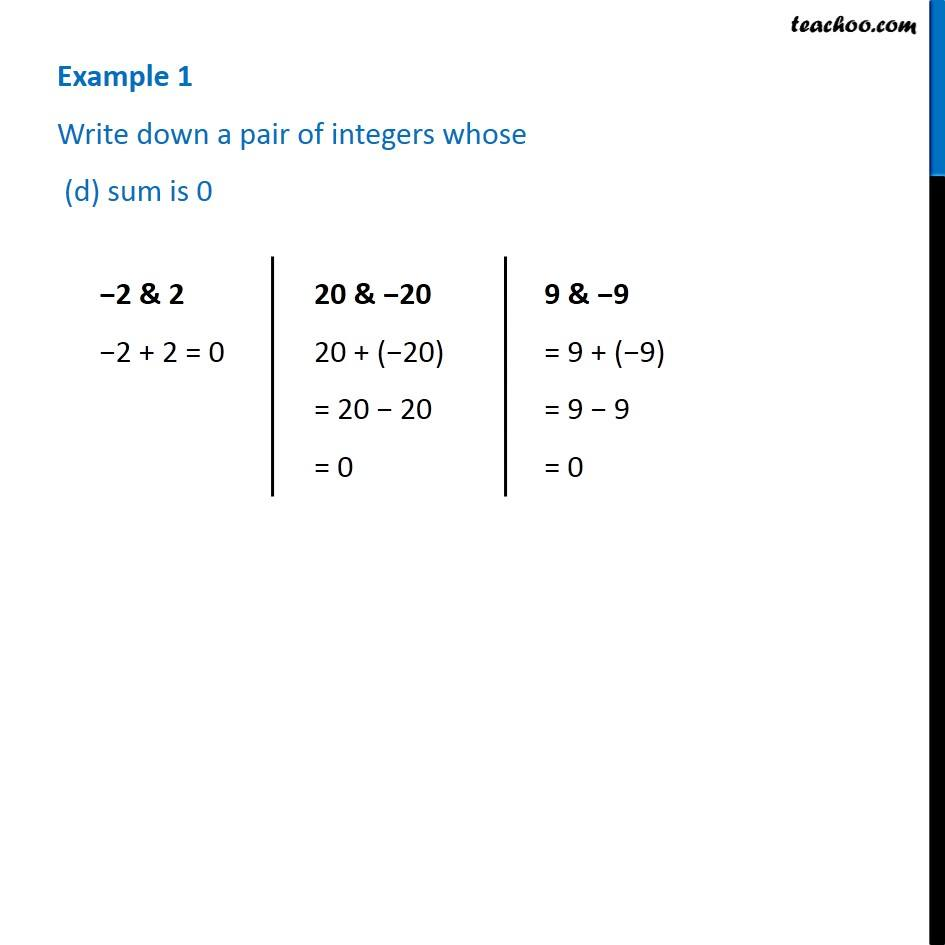Subscribe to our Youtube Channel - https://you.tube/teachoo

1. Chapter 1 Class 7 Integers
2. Serial order wise
3. Examples

Transcript

Example 1 Write down a pair of integers whose (a) sum is –3 −3 & 0 −3 + 0 = −3 −1 & −2 −1 + (−2) = −1 − 2 = −(1 + 2) = −3 2 & −5 2 + (−5) = 2 − 5 = –(–2 + 5) = –(5 – 2) = −3 2 & −5 2 + (−5) = 2 − 5 = –(–2 + 5) = –(5 – 2) = −3 Example 1 Write down a pair of integers whose (b) difference is –5 −5 & 0 −5 − 0 = −5 −2 & 3 −2 − (3) = −2 − 3 = −(2 + 3) = −5 3 & 8 3 − 8 = −(−3 + 8) = −(8 − 3) = −5 Example 1 Write down a pair of integers whose (c) difference is 2 2 & 0 2 − 0 = 2 5 & 3 5 − 3 = 2 1 & –1 1 − (−1) = 1 + 1 = 2 Example 1 Write down a pair of integers whose (d) sum is 0−2 & 2 −2 + 2 = 0 20 & −20 20 + (−20) = 20 − 20 = 0 9 & −9 = 9 + (−9) = 9 − 9 = 0

Examples

Chapter 1 Class 7 Integers
Serial order wise